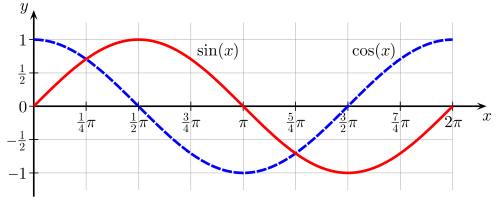# One condition: No calculator!

If $R = \sin 130^\circ+\cos 130^\circ$, then which of the following is true?×